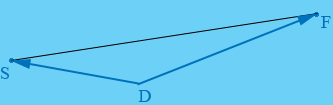SEARCH HOMEMath Central Quandaries & QueriesQuestion from dom, a student: Two boats leave port at the same time. They leave at 150 degree angle. One boat travels at 10mph and the other at 20mph. After two hours how far are the boats apart?Dom,In my diagram D is the dock where the boat started, S is the position of the slop boat after 2 hours and F is the position of the fast boat after 2 hours.

The slow boat travelled at 10 mph for 2 hours. How far did it travel?
The fast boat travelled at 20 mph for 2 hours. How far did it travel?

The angle SDF has a measure of 150o and you want to know the distance from F to S. This looks like a job for the Law of Cosines.

PennyMath Central is supported by the University of Regina and The Pacific Institute for the Mathematical Sciences.